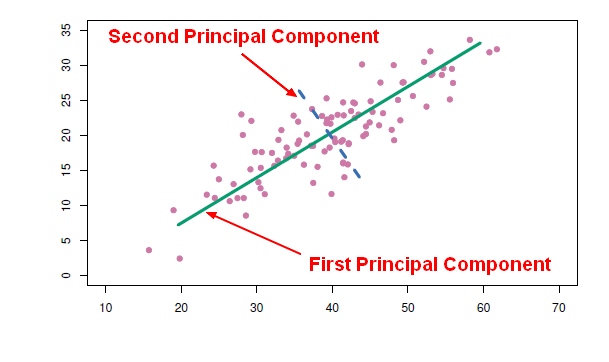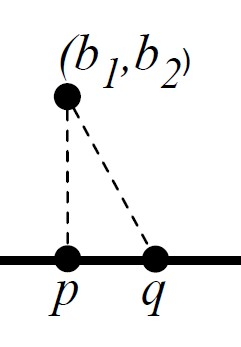# Linear Algebra - Orthogonality (Perpendicular)

Orthogonal is linear-algebra-ese for perpendicular.

## Definition

The squared length of the “hypotenuse” (the vector u + v) is

$\begin{array}{rcll} {\left \| u + v \right \|}^2 & = & \left \langle u+v,u+v \right \rangle & \\ & = & \left \langle u,u+v \right \rangle + \left \langle v,u+v \right \rangle & \text{by linearity of inner product} \\ & = & \left \langle u,u \right \rangle + \left \langle u,v \right \rangle + \left \langle v,u \right \rangle + \left \langle v,v \right \rangle & \text{by symmetry and linearity of inner product} \\ & = & {\left \| u \right \|}^2 + 2 \left \langle u,v \right \rangle + {\left \| v \right \|}^2 & \text{by symmetry of inner product} \\ \end{array}$

Thus following the Pythagorean Theorem u and v are orthogonal when $$\left \langle u,v \right \rangle = 0$$

then:

$${\left \| u + v \right \|}^2 = {\left \| u \right \|}^2 + {\left \| v \right \|}^2$$

• every vector v is orthogonal to u when u is the zero vector.

## Property

• Property O1: If u is orthogonal to v then u is orthogonal to $\alpha v$ for every scalar $\alpha$ .
• Property O2: If u and v are both orthogonal to w then u + v is orthogonal to w.

## Example

• [1, 2] · [2,-1] = 0 so [1, 2] · [20,-10] = 0
• Second Example:
[1, 2, 1]       . [1,-1, 1] = 0
[0, 1, 1]       . [1,-1, 1] = 0
----------------------------------------
([1, 2, 1] + [0, 1, 1]) . [1,-1, 1] = 0



## Type

### Mutual

$v_1, \dots, v_n$ are mutually orthogonal if $v_i$ is orthogonal to $v_j$ for every pair $i,j$ such that $i \neq j$ .

Example: $[1, 2, 1], [1,−1, 1], [1, 0,−1]$ are mutually orthogonal: $$\begin{array}{rrrr} \langle & [1, 2, 1], & [1,−1, 1] & \rangle & = & 0 \\ \langle & [1, 2, 1], & [1, 0,−1] & \rangle & = & 0 \\ \langle & [1,−1, 1], & [1, 0,−1] & \rangle & = & 0 \end{array}$$

Lemma: If $v_1, \dots, v_n$ are mutually orthogonal then, for any scalars $\alpha_1, \dots, \alpha_n$ : $${\| \alpha_1.v_1, \dots, \alpha_n.v_n \|}^2 = {\alpha_1}^2.{\| v_1 \|}^2 + \dots + {\alpha_n}^2.{\| v_n \|}^2$$

#### Mutually orthogonal nonzero are independent

Mutually orthogonal nonzero vectors are linearly independent

Proof: Let $v_1^* , \dots , v_n^*$ be mutually orthogonal nonzero vectors, then they are linearly independent if the coefficient $\alpha_0, \alpha_1 , \dots , \alpha_n$ are null in the following statement: $$0 = \alpha_0 v_0^* + \alpha_1 v_1^* + \dots + \alpha_n v_n^*$$

To show that $\alpha_0$ is zero, take inner product with $v_0^*$ on both sides:

$$\begin{array}{rrl} \langle v_0^*, 0 \rangle & = & \langle v_0^*, \alpha_0 v_0^* + \alpha_1 v_1^* + \dots + \alpha_n v_n^* \rangle \rangle \\ 0 & = & \alpha_0 \langle v_0^*, v_0^* \rangle + \alpha_1 \langle v_0^*, v_1^* \rangle + \dots + \alpha_n \langle v_0^*, v_n^* \rangle \\ 0 & = & \alpha_0 {\| v_0^* \|}^2 + \alpha_1 0 + \dots + \alpha_n 0 \\ 0 & = & \alpha_0 {\| v_0^* \|}^2 \\ 0 & = & \alpha_0 {\| v_0^* \|}^2 \\ \end{array}$$ Since $v_0^*$ is nonzero, the only solution is $\alpha_0 = 0$

## Operations

### Scaling

Scaling orthogonal vectors gives orthogonal vectors. If u is orthogonal to v then, for any scalars $\alpha, \beta$ : $${\left \|\alpha u + \beta v \right \|}^2 = \alpha^2 {\left \|\alpha u \right \| }^2 + \beta^2 {\left \|\alpha v \right \| }^2$$

Discover MoreData Mining - Principal Component (Analysis|Regression) (PCA|PCR)

Principal Component Analysis (PCA) is a feature extraction method that use orthogonal linear projections to capture the underlying variance of the data. By far, the most famous dimension reduction approach...Linear Algebra - Basis of a Vector Space

A basis for vector space V is a linearly independent set of generators for V. Thus a set S of vectors of V is a basis for V if S satisfies two properties: Property B1 (Spanning) Span S = V, and Property...Linear Algebra - Closest Point on a Line

How to find the closest point on a line from a point ? How to find the vector on the line that best approximates the given vector b (the closest point on the line) Letb be a vector Leta be a...Linear Algebra - Closest point in higher dimension than a plane

Solving closest point in the span of many vectors Goal: An algorithm that, given a vector b and vectors v1, . . . , vn, finds the vector in Span {v1, . . . , vn} that is closest to b. Special case:...Linear Algebra - Find a basis computation problem

Find a basis for a vector space Example: Find a basis for the null space of By the dot-product definition of matrix-vector multiplication, a vector v is in the null space of A if the dot-product...Linear Algebra - Generators of a Vector Space

The generators for the set of vectors are the vectors in the following formula: where is a generating set for {[3, 0, 0], [0, 2, 0], [0, 0, 1]} is a generating set for . = Span {[3, 0,...Linear Algebra - Inner product of two vectors

Inner products allow the rigorous introduction of intuitive geometrical notions such as the length of a vector or the angle between two vectors. They also provide the means of defining orthogonality between...Linear Algebra - Orthogonal complement Vector Space

Let U be a of W. For each vector b in W, we can write b as the following projections: where: is in U, and is orthogonal to every vector in U. Let V be the set . V is the orthogonal complement...Linear Algebra - Orthogonalization - Building an orthogonal set of generators

Original stated goal: Find the projection of b orthogonal to the space V spanned by arbitrary vectors Input: A list of vectors over the reals output: A list of mutually orthogonal vectors such...Linear Algebra - Pythagorean Theorem

Following the definition of orthogonality, the Pythagorean Theorem for vectors becomes: